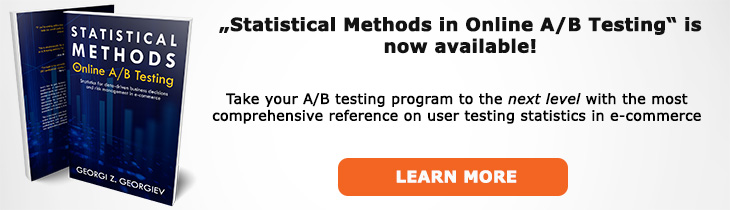# What does "Two-Tailed Test" mean?

Definition of Two-Tailed Test in the context of A/B testing (online controlled experiments).

## What is a Two-Tailed Test?

Aliases: two-sided test

A two-tailed test in hypothesis testing is a test defined so that it matches a two-sided hypothesis. The "tail" references one half of the frequency distribution of the statistic of interest (e.g. a z score) and a test having two tails refers to the fact that the rejection region is split on two sides of the distribution mean. The term "two-sided test" is recommended to avoid confusion due to misconceptions about the relationship between the tails of a distribution and the sided-ness of a hypothesis.

If the distribution of the statistic is symmetrical one can easily convert the result from a one-tailed test to a two-tailed one by multiplying the p-value by 2 or by considering the confidence level of an interval to be (100-(100-XX%)*2)% where XX% is the confidence level of a one-tailed interval.

Since in an A/B test the hypothesis of interest is usually one-sided we rarely, if ever, need to perform two-sided tests.

## Articles on Two-Tailed Test

Like this glossary entry? For an in-depth and comprehensive reading on A/B testing stats, check out the book "Statistical Methods in Online A/B Testing" by the author of this glossary, Georgi Georgiev.## Glossary Index by Letter

Select a letter to see all A/B testing terms starting with that letter or visit the Glossary homepage to see all.